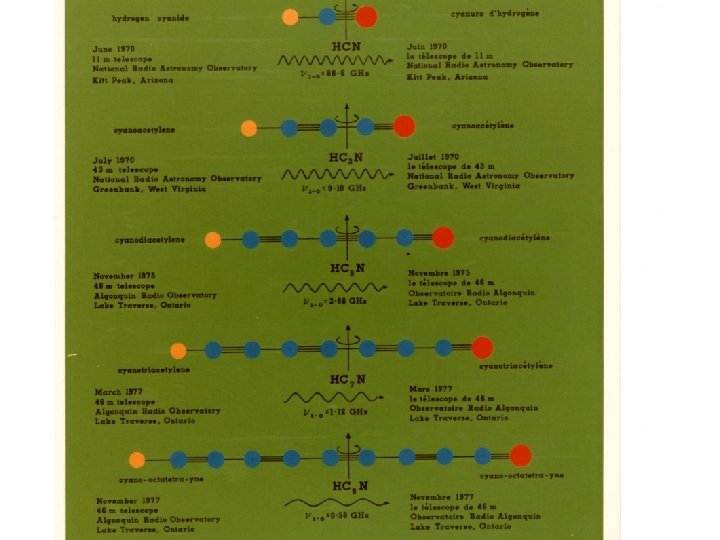Скачать презентацию CH and DIBs toward Herschel 36 Takeshi Oka

f032d028f66de48ec4911f39f2ab0000.ppt

• Количество слайдов: 11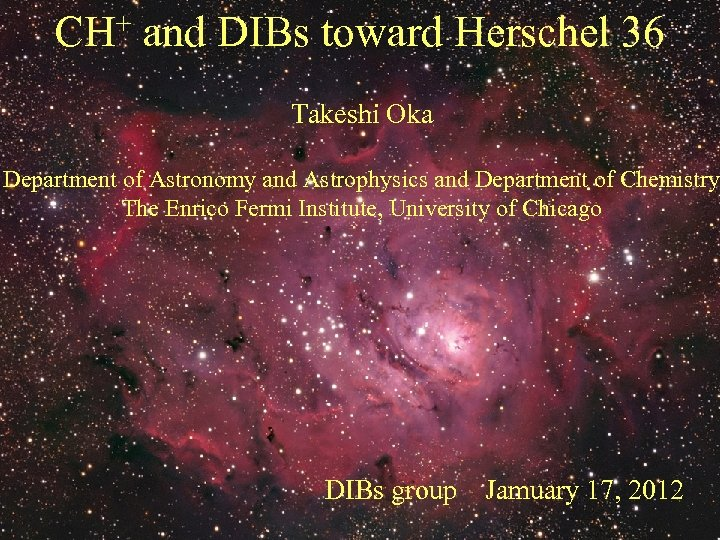CH+ and DIBs toward Herschel 36 Takeshi Oka Department of Astronomy and Astrophysics and Department of Chemistry The Enrico Fermi Institute, University of Chicago DIBs group Jamuary 17, 2012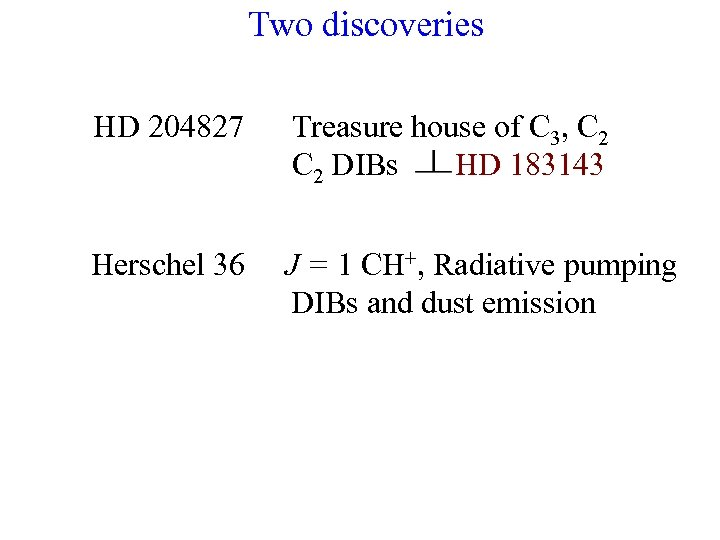Two discoveries HD 204827 Treasure house of C 3, C 2 DIBs HD 183143 Herschel 36 J = 1 CH+, Radiative pumping DIBs and dust emission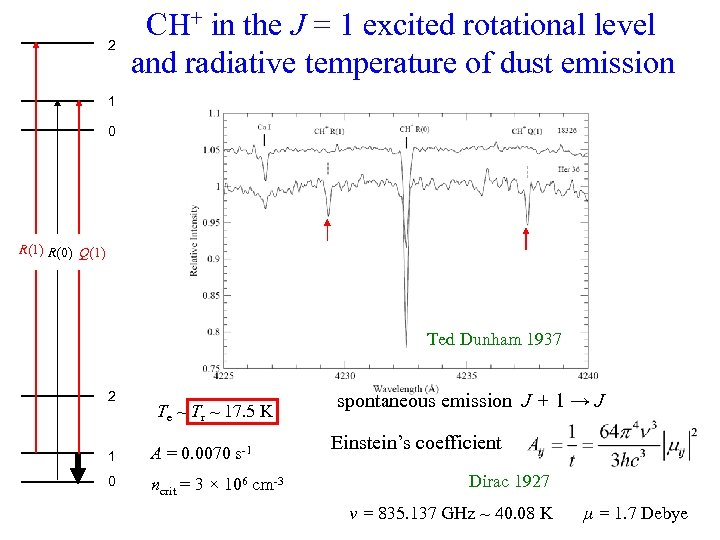2 CH+ in the J = 1 excited rotational level and radiative temperature of dust emission 1 0 R(1) R(0) Q(1) Ted Dunham 1937 2 Te ~ Tr ~ 17. 5 K 1 A = 0. 0070 s-1 0 ncrit = 3 × 106 cm-3 spontaneous emission J + 1 → J Einstein’s coefficient Dirac 1927 ν = 835. 137 GHz ~ 40. 08 K μ = 1. 7 Debye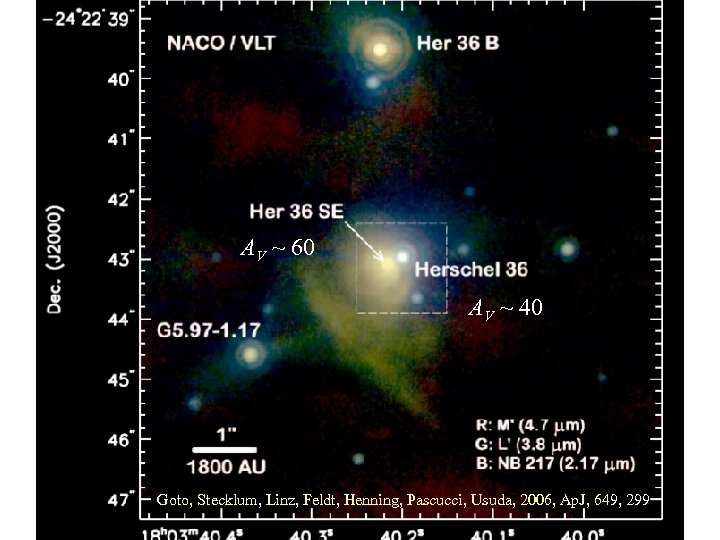AV ~ 60 AV ~ 40 Goto, Stecklum, Linz, Feldt, Henning, Pascucci, Usuda, 2006, Ap. J, 649, 299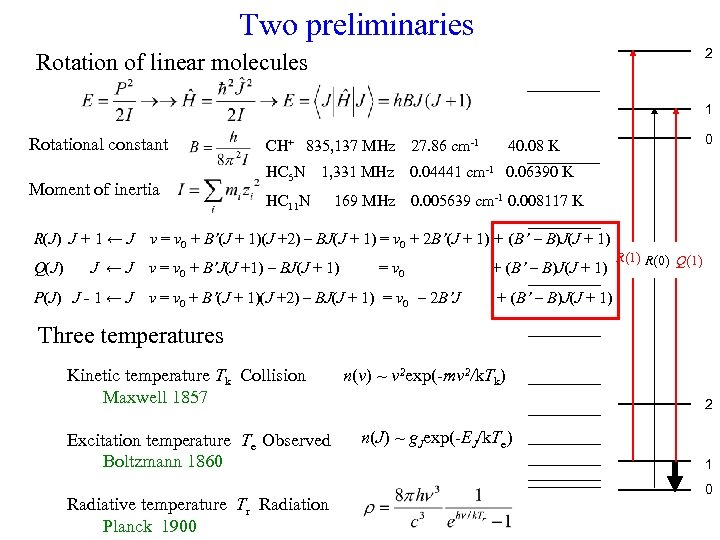Two preliminaries 2 Rotation of linear molecules 1 Rotational constant Moment of inertia CH+ 835, 137 MHz 27. 86 cm-1 HC 5 N 1, 331 MHz 0. 04441 cm-1 0. 06390 K HC 11 N 0. 005639 cm-1 0. 008117 K 169 MHz R(J) J + 1 ← J ν = ν 0 + B’(J + 1)(J +2) – BJ(J + 1) = ν 0 + 2 B’(J + 1) + (B’ – B)J(J + 1) Q(J) ν = ν 0 + B’J(J +1) – BJ(J + 1) J ←J P(J) J ˗ 1 ← J 0 40. 08 K = ν 0 ν = ν 0 + B’(J + 1)(J +2) – BJ(J + 1) = ν 0 – 2 B’J + (B’ – B)J(J + 1) R(0) Q(1) + (B’ – B)J(J + 1) Three temperatures Kinetic temperature Tk Collision Maxwell 1857 Excitation temperature Te Observed Boltzmann 1860 Radiative temperature Tr Radiation Planck 1900 n(v) ~ v 2 exp(-mv 2/k. Tk) 2 n(J) ~ g. Jexp(-EJ/k. Te) 1 0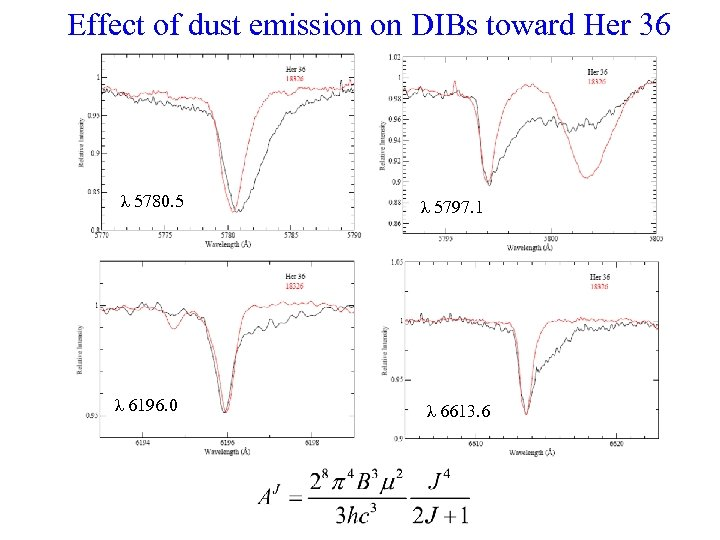Effect of dust emission on DIBs toward Her 36 λ 5780. 5 λ 6196. 0 λ 5797. 1 λ 6613. 6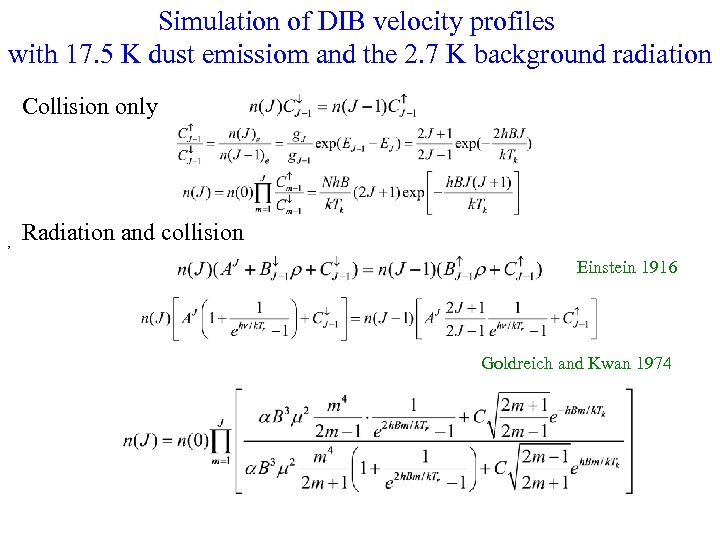Simulation of DIB velocity profiles with 17. 5 K dust emissiom and the 2. 7 K background radiation Collision only , Radiation and collision Einstein 1916 Goldreich and Kwan 1974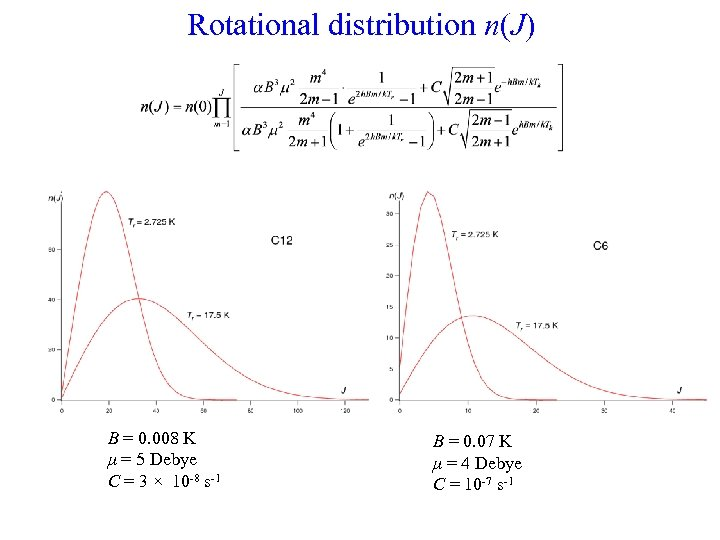Rotational distribution n(J) B = 0. 008 K μ = 5 Debye C = 3 × 10 -8 s-1 B = 0. 07 K μ = 4 Debye C = 10 -7 s-1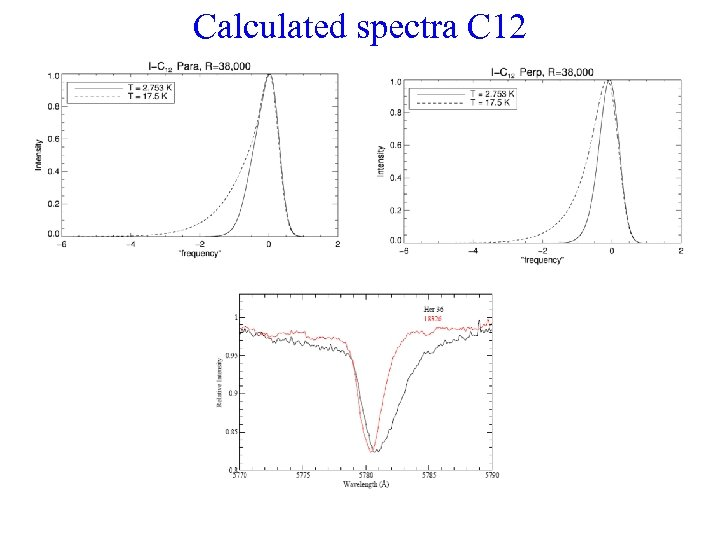Calculated spectra C 12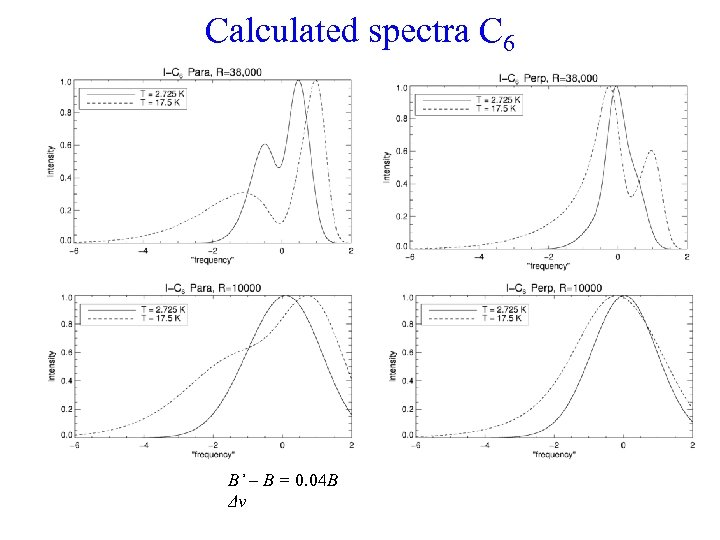Calculated spectra C 6 B’ – B = 0. 04 B Δν